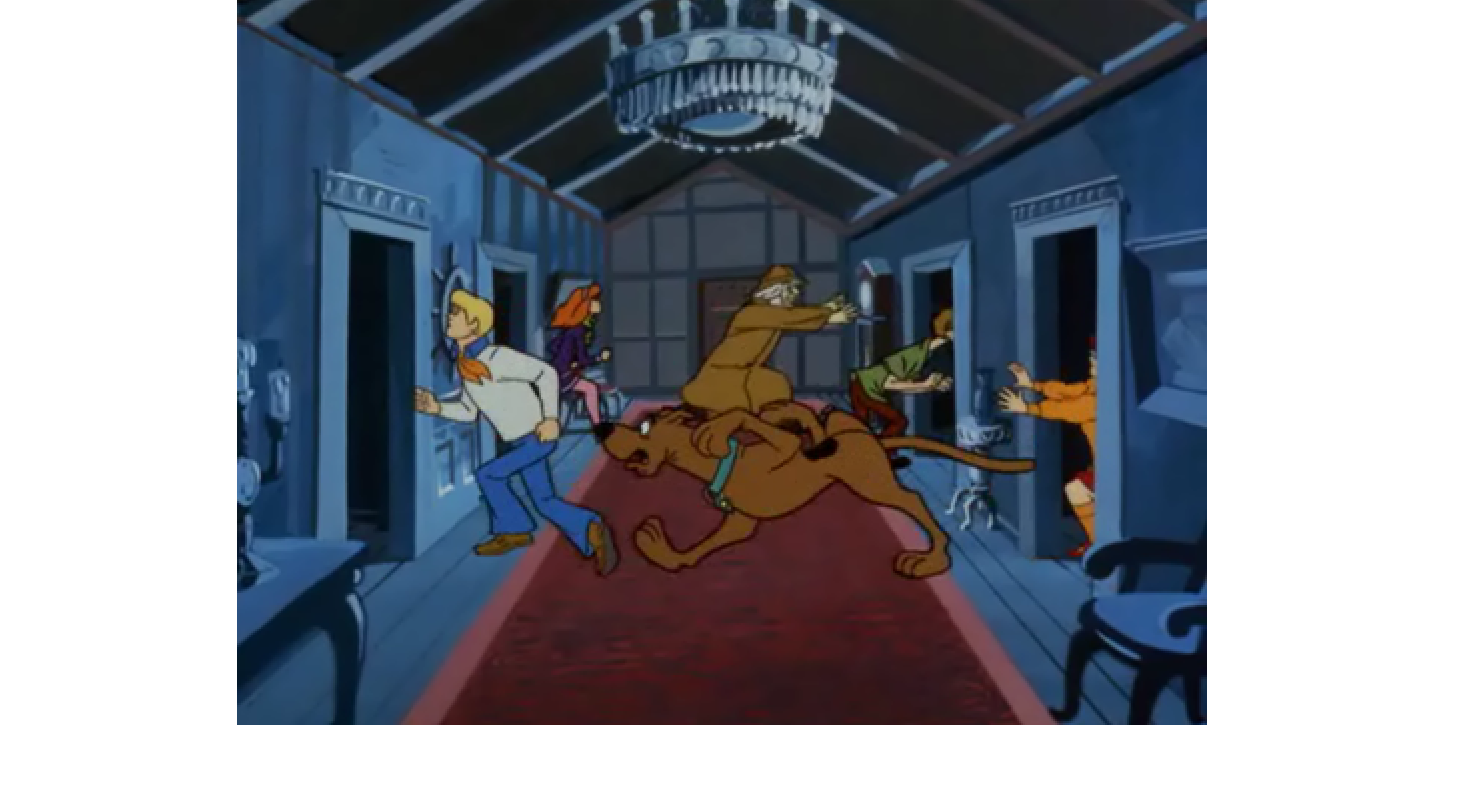# Run & HideThere are 4 human friends of the Mystery Inc. (assuming Scooby-Doo is always with Shaggy) running away from a mysterious ghost, and there are 4 available rooms to hide in.

Any of these rooms can contain up to 4 teenagers (or none at all).

If the ghost opens one of these 4 rooms in random, what is the probability that he will see no-one hiding in there?

If this probability can be expressed as $\dfrac{a}{b}$, where $a$ and $b$ are coprime positive integers, enter $a+b$ as your answer.

×